The FACTOR Procedure
 Time Requirements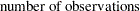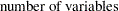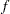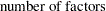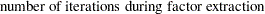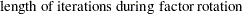The time required to compute... is roughly proportional to an overall factor analysis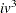the correlation matrix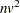PRIORS=SMC or ASMC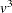PRIORS=MAX
eigenvaluesfinal eigenvectors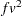generalized Crawford-Ferguson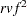family of rotations, PROMAX, or HK ROTATE=PROCRUSTES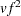Each iteration in the PRINIT or ALPHA method requires computation of eigenvalues and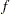eigenvectors.

Each iteration in the ML or ULS method requires computation of eigenvalues and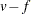eigenvectors.

The amount of time that PROC FACTOR takes is roughly proportional to the cube of the number of variables. Factoring 100 variables, therefore, takes about 1000 times as long as factoring 10 variables. Iterative methods (PRINIT, ALPHA, ULS, ML) can also take 100 times as long as noniterative methods (PRINCIPAL, IMAGE, HARRIS).Previous Page | Next Page | Top of Page